# Changing dot type, dot size, and line type

Several customizable plotting options include

• Changing the point shape using `pch=` (options are 0 through 25)
• Changing the point size using `cex=`
• Changing the line type for lines using `lty=` (options are 0 through 6)

Example for changing shape
There are 26 available shapes which can be specified (default is 1 a hollow dot)

``plot(0:25, pch = 0:25)``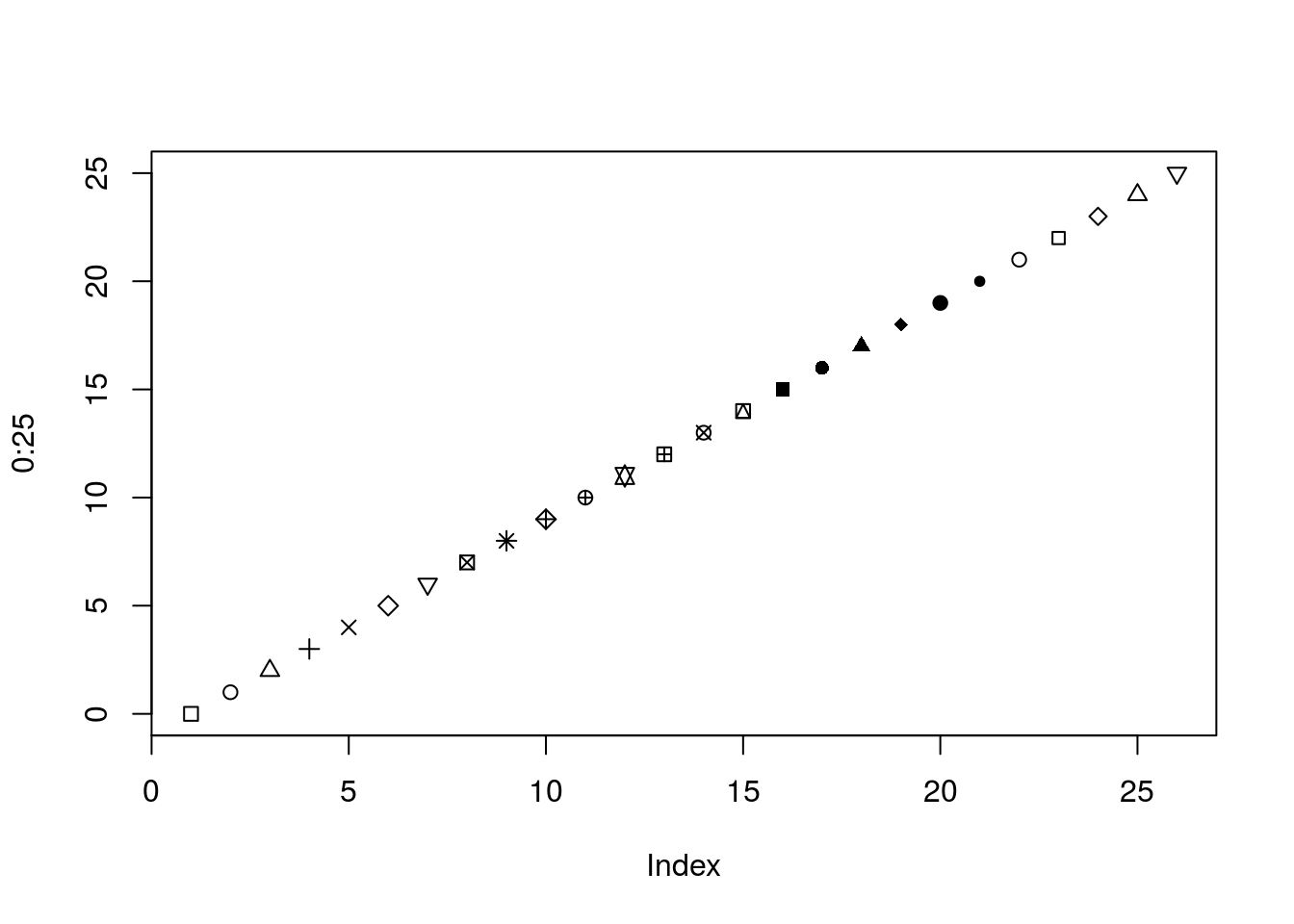Also for the shapes 21-25 the `col=` parameter changes the border color and the `bg=` changes the inner color

``plot(0:25, pch = 0:25, col = "red", bg = "darkblue")``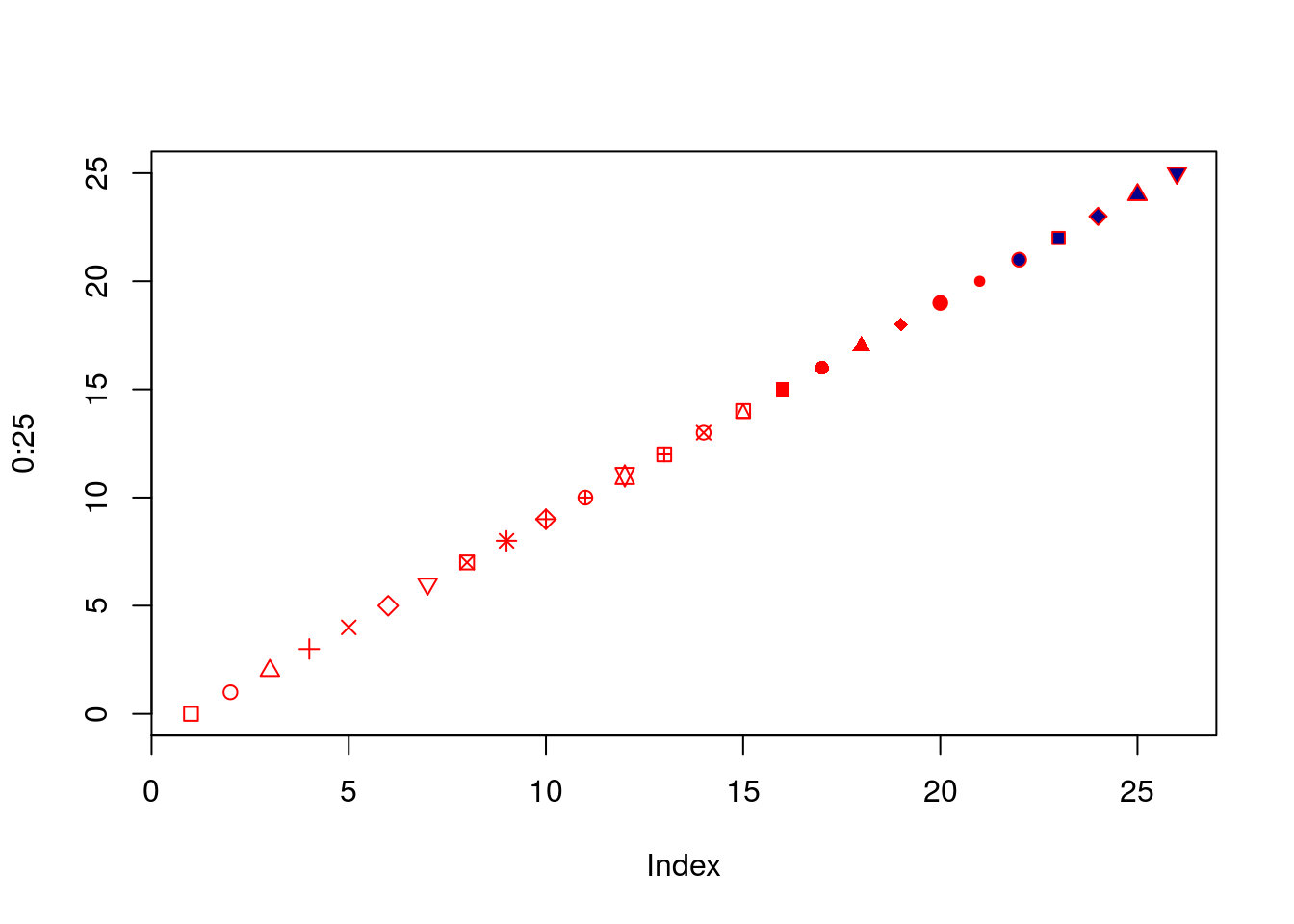You can also change the size of dots by using `cex=`. You give it a relative value, something that has a `cex` value of 4 will be twice as big as something that has a `cex` value of 2, but the number itself doesn’t mean a size

``plot(1:10, cex = 1:10)``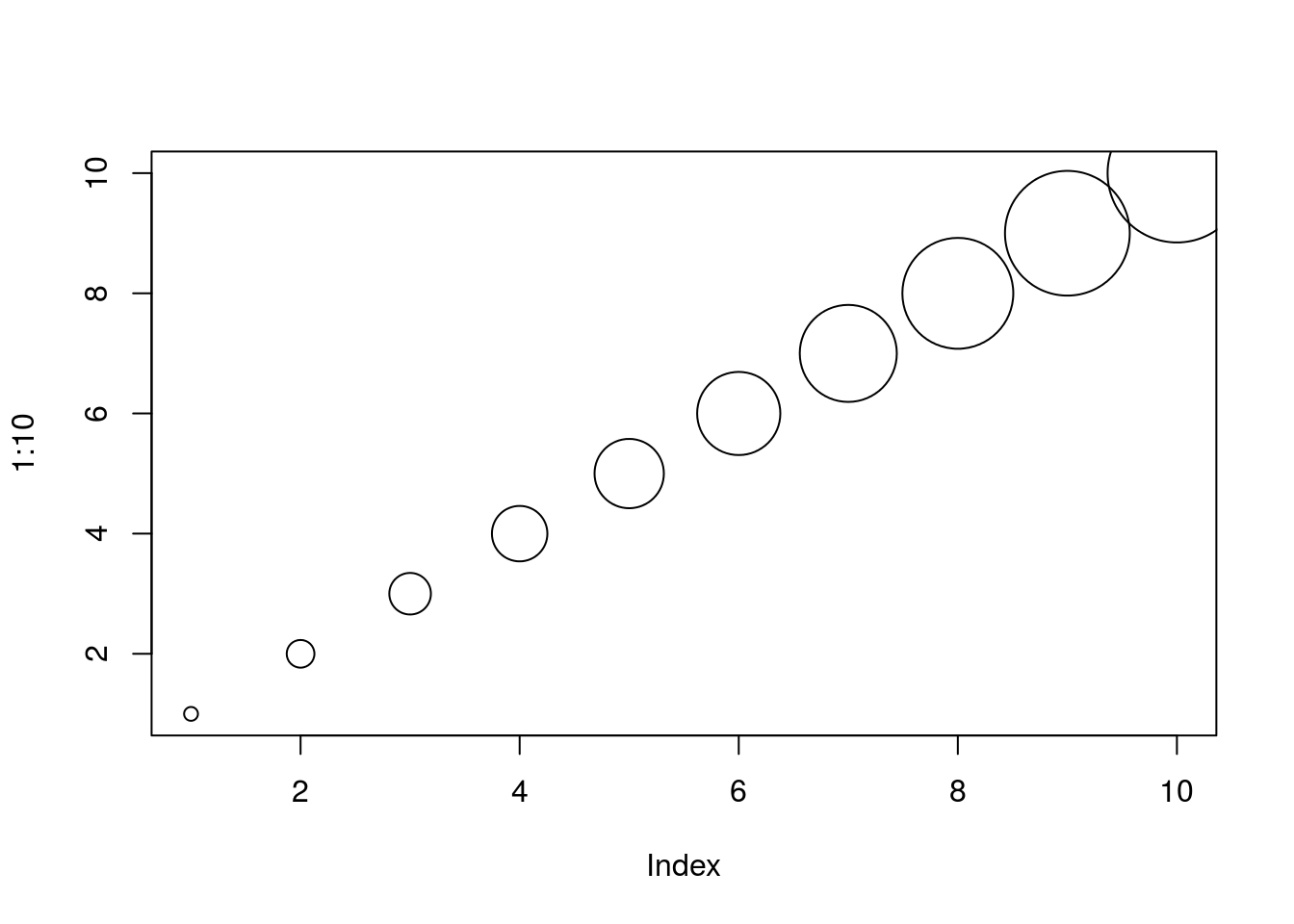When doing line plots, you can change the type of line being drawn by using `lty=` There are seven options given by giving lty 0 through 6

number type of line
0 blank
1 solid
2 dashed
3 dotted
4 dotdash
5 longdash
6 twodash
``````# blank you can't see it
plot(c(0,1), c(0,0), ylim = c(0,6), type="l", lty=0)
# solid (the default)
lines(c(0,1), c(1,1), type="l", lty=1)
# dashed
lines(c(0,1), c(2,2), type="l", lty=2)
# dotted
lines(c(0,1), c(3,3), type="l", lty=3)
# dotdash
lines(c(0,1), c(4,4), type="l", lty=4)
# longdash
lines(c(0,1), c(5,5), type="l", lty=5)
# twodash
lines(c(0,1), c(6,6), type="l", lty=6)``````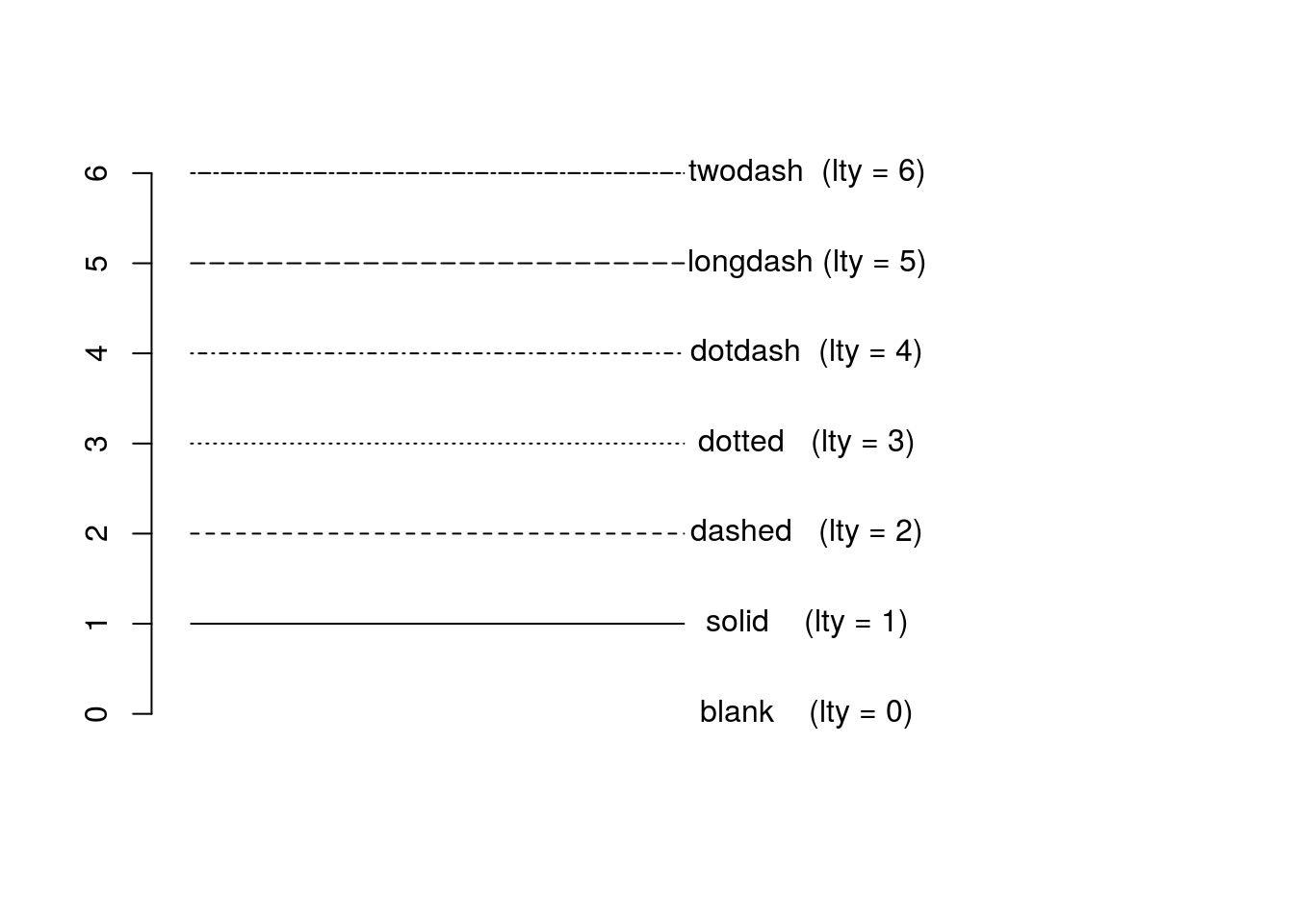# Using logic to help with plotting

A helpful to add to a plot is the `ifelse()` function. You give it three things

1. A vector of TRUEs and FALSEs
2. A value for TRUE
3. A value for FALSE

What this function does is return a new vector by replacing wherever there are TRUEs in the input with the value for TRUE and wherever there are FALSEs with the value for FALSE

For example

``ifelse(c(TRUE,FALSE,TRUE, FALSE), "Replace_TRUE", "Replace_FALSE")``
`` "Replace_TRUE"  "Replace_FALSE" "Replace_TRUE"  "Replace_FALSE"``

This can also work by giving it a logic test on a vector (which will be converted into a vector of TRUEs and FALSEs anyways)

``````testNumbers = c(1,2,3,4)

testNumbers > 2``````
`` FALSE FALSE  TRUE  TRUE``
``ifelse(testNumbers >2, "Greater_than_two", "Less_than_two")``
`` "Less_than_two"    "Less_than_two"    "Greater_than_two" "Greater_than_two"``

And you can of course combine logic tests

``ifelse(testNumbers >2 & testNumbers < 4, "Greater_than_two_less_than_four", "Less_than_two_or_greater_than_four")``
`````` "Less_than_two_or_greater_than_four" "Less_than_two_or_greater_than_four"
 "Greater_than_two_less_than_four"    "Less_than_two_or_greater_than_four"``````

This function can be helpful for change colors or shapes of scatter plots to demonstrate thresholds

For example lets look at a scatter plot of 100 randomly generated points with x ranging from -1 to 1 and y ranging from -1 to 1

``````testNumberData = data.frame(x = runif(100,-1,1), y = runif(100,-1,1))

plot(testNumberData\$x, testNumberData\$y)

# add a line for x = 0 and y = 0

abline(v = 0)

abline(h = 0)``````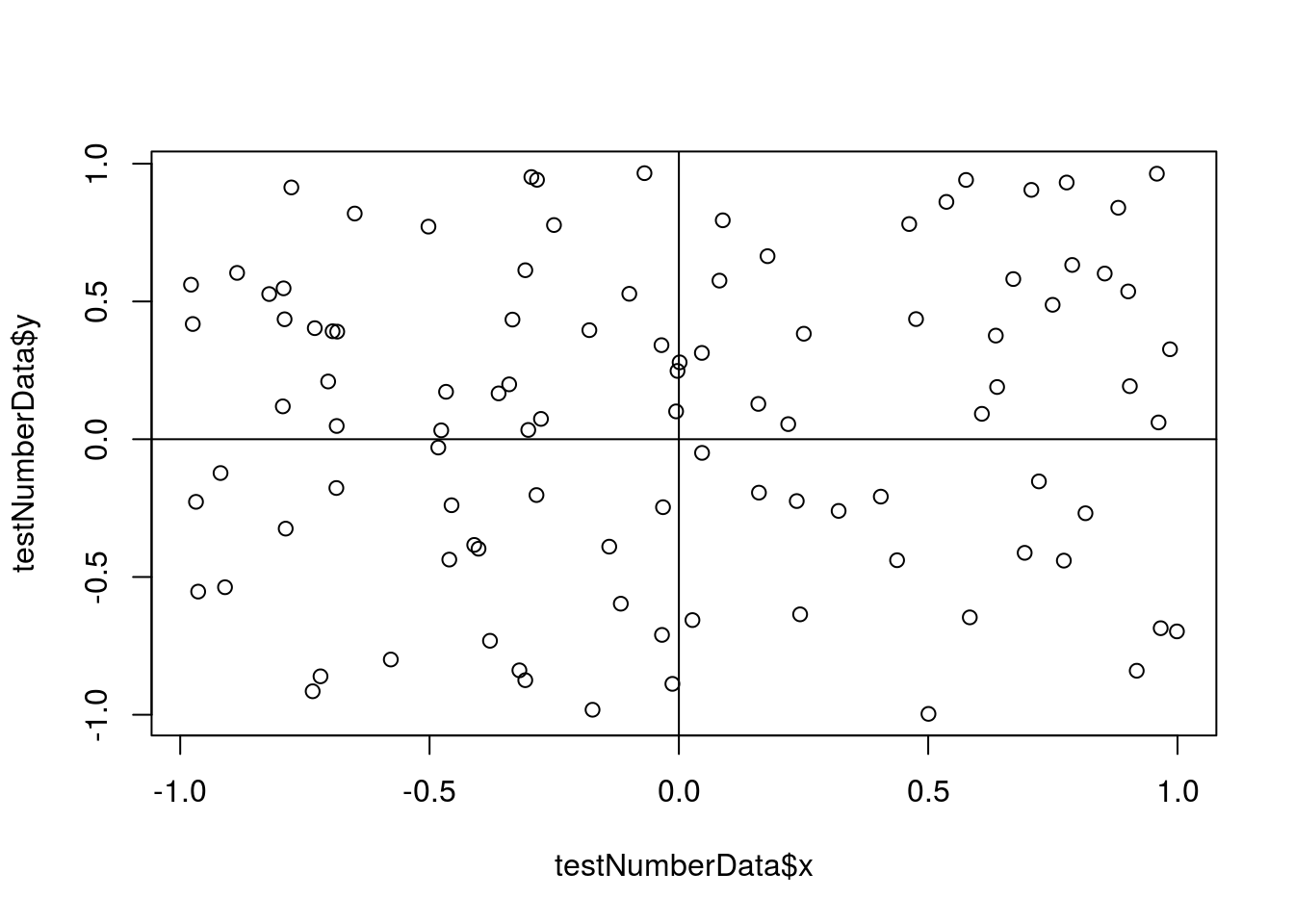If you were interested in marking points that have a positive x value you could use `ifelse()` with `col` along with the package RColorBrewer to color the points differently

``install.packages("RColorBrewer")``
``````library(RColorBrewer)
cols = brewer.pal(2, "Dark2")

plot(testNumberData\$x, testNumberData\$y,
col = ifelse(testNumberData\$x > 0, cols, cols))

# add a line for x = 0 and y = 0

abline(v = 0)

abline(h = 0)``````